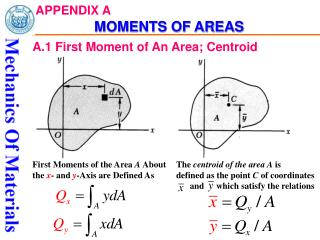DownloadDownload PresentationAPPENDIX A MOMENTS OF AREAS

# APPENDIX A MOMENTS OF AREAS

Télécharger la présentation## APPENDIX A MOMENTS OF AREAS

- - - - - - - - - - - - - - - - - - - - - - - - - - - E N D - - - - - - - - - - - - - - - - - - - - - - - - - - -
##### Presentation Transcript

1. APPENDIX AMOMENTS OF AREAS A.1 First Moment of An Area; Centroid First Moments of the Area A About the x- and y-Axis are Defined As The centroid of the area A is defined as the point C of coordinates and which satisfy the relations

2. APPENDIX AMOMENTS OF AREAS The first moment of an area about its symmetric axis is zero, so, the centroid of the area must be on the symmetric axis. When an area possesses a center of symmetry O, the first moment of the area about any axis through O is zero. In other words, O is the centroid of the area.

3. APPENDIX AMOMENTS OF AREAS If an area has two symmetric axes, the inter-section of the two axes must be the centroid of the area.

4. APPENDIX AMOMENTS OF AREAS Sample Problem A.1 For the triangular area of Fig. (a), determine (a) the first moment Qx of the area with respect to the x-axis, (b) the coordinate of the centroid of the area. Fig. (a) Fig. (b)

5. APPENDIX AMOMENTS OF AREAS dA=udy,

6. APPENDIX AMOMENTS OF AREAS Complement Problem Determine the first moment of a semi-circular area about the x-axis, (b) the coordinate of the centroid of the semi-circle.

7. APPENDIX AMOMENTS OF AREAS A.1 Determination of The First Moment And Centroid of A Composite Area

8. APPENDIX AMOMENTS OF AREAS Sample Problem A.2 Locate the Centroid C of the area A shown in Fig. (a). Fig. (a) Fig. (b)

9. APPENDIX AMOMENTS OF AREAS Solution: A1=80×20=1600 mm2, A2=60×40=2400 mm2

10. APPENDIX AMOMENTS OF AREAS Sample Problem A.3 Referring to the area A of Sample Problem A.2, we consider the horizontal x axis which is through its centroid C. (Such an axis is called a centroidal axis.) Denoting by Athe portion of A located above that axis (Fig. a), determine the first moment of A with respect to the x axes. Fig. (a) Fig. (b) Fig. (c)

11. APPENDIX AMOMENTS OF AREAS Solution: 0 In fact

12. y C2 c(19.7;39.7) C1 x Complement Problem Determine the centroid of the L-shape area. 10 10 80

13. 10 y 120 10 80 x Alternative method: Negative area method x

14. APPENDIX AMOMENTS OF AREAS A.3 Second Moment, or Moment of Inertia, of An Area; Radius of Gyration Moment of Inertia of A With Respect To the And x Axis And y Axis are Defined, Respectively, As Define the Polar Moment of Inertia of the Area A With Respect To Point O As the Integral :

15. APPENDIX AMOMENTS OF AREAS Radii of Gyration of An Area Awith respect to the x and y axis: Radii of Gyration With Respect To the Origin O

16. APPENDIX AMOMENTS OF AREAS Sample Problem A.4 For the rectangular area of Fig.(a). determine (a) the moment of inertia Ix of the area with respect to the centroidal x axis. (b) the corresponding radius of gyration rx. Fig. (b) Fig. (a)

17. APPENDIX AMOMENTS OF AREAS Sample Problem A.5 For the circular area of Fig. (a), determine (a) the polar moment of inertia JO, (b) rectangular moments of inertia Ix and Iy. Fig. (a) Fig. (b)

18. APPENDIX AMOMENTS OF AREAS A.4 Parallel-Axis Theorem

19. APPENDIX AMOMENTS OF AREAS A.5 Determination of The Moment of Inertia of a Composite Area.

20. APPENDIX AMOMENTS OF AREAS Sample Problem A.6 Determine the moment of inertia of the area shown with respect to the centroidal x axis (Fig. a). Fig. (a) Fig. (b)

21. APPENDIX AMOMENTS OF AREAS Solution:

22. APPENDIX AMOMENTS OF AREAS A.6 Product of Inertia for An Area. product of inertia for an element of area located at point (x, y)is defined as If either x or y axis is a symmetric axis, Ixy=0.

23. APPENDIX AMOMENTS OF AREAS Parallel-Axis theorem

24. APPENDIX AMOMENTS OF AREAS Sample Problem A.7 Determine the product of inertia Ixy of the triangle shown in Fig. (a).

25. APPENDIX AMOMENTS OF AREAS Sample Problem A.7

26. APPENDIX AMOMENTS OF AREAS Solution:

27. APPENDIX AMOMENTS OF AREAS Sample Problem A.8 Compute the product of inertia of the beam’s cross-sectional area, shown in Fig. (a), about the x and y centroidal axes.

28. APPENDIX AMOMENTS OF AREAS Solution: Rectangle A mm4 Rectangle B Rectangle D mm4 mm4 The product of inertia for the entire cross section is mm4

29. APPENDIX AMOMENTS OF AREAS A.7 Moments of Inertia for An Area About Inclined Axes

30. APPENDIX AMOMENTS OF AREAS

31. APPENDIX AMOMENTS OF AREAS Principal Moments of Inertia

32. APPENDIX AMOMENTS OF AREAS

33. APPENDIX AMOMENTS OF AREAS Sample Problem A.9 Determine the principal moments of inertia for the beam’s cross-sectional area shown in Fig. (a)with respect to an axis passing through the centroid.

34. APPENDIX AMOMENTS OF AREAS A.8 Mohr’s Circle for Moments of Inertia

35. APPENDIX AMOMENTS OF AREAS Sample Problem A.10 Using Mohr’s circle, determine the principal moments of inertia for the beam’s cross-sectional area, shown in Fig. (a), with respect to an axis passing through the centroid.

36. APPENDIX AMOMENTS OF AREAS